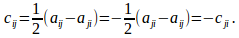## Proof That Any Second Order System Can Be Written as a Sum or Second Order Symmetric and Skew Symmetric Systems

Theorem

Any second order system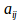can be written as a sum of a second order symmetric system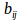satisfying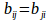and a second order skew symmetric system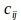satisfying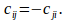Proof

For eachwrite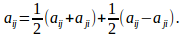Then write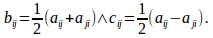Then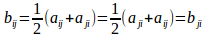and